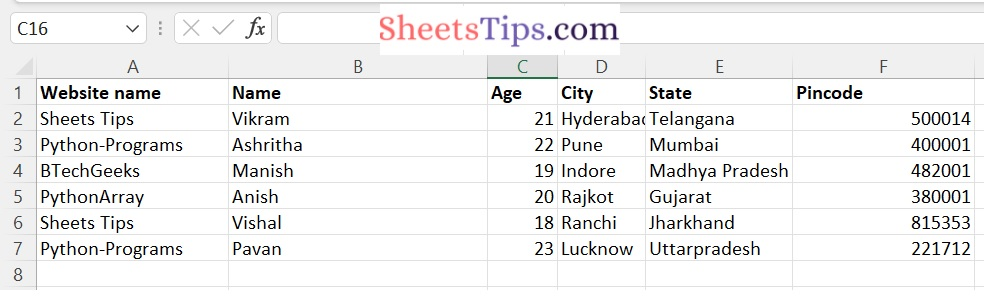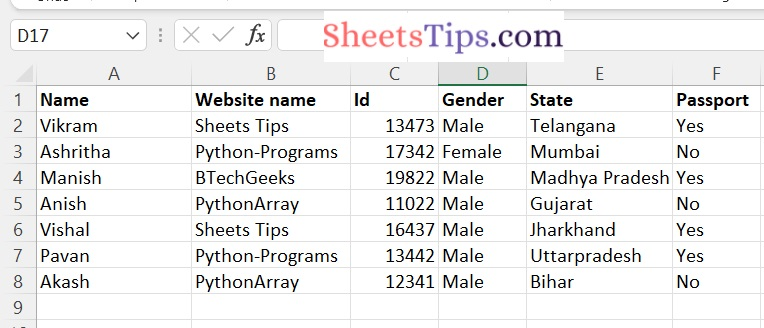# Python Program to Compare Excel Files

In this article, we are going to compare the two excel files columns in Python and their column numbers respectively.

Prerequisites:

## Program to Compare Excel Files in Python

sampleExcelFile1.xlsx:

This file contains 6 columns with 6 unique student details. The following are the column names:

• Website name
• Name
• Age
• City
• State
• PincodesampleExcelFile2.xlsx:

This file contains 6 columns with 7 unique student details. The following are the column names:

• Name
• Website name
• Id
• Gender
• State
• PassportThe read excel method accepts two arguments: sheet name and index col. sheet name defines the sheet from which the data frame should be created, and the index col gives the title column.

Approach:

• Import the pandas module using the import Keyword.
• Read the first excel file using the read_excel() function by passing the argument file path of the first excel file and store it in a variable.
• Read the second excel file using the read_excel() function by passing the argument file path of the second excel file and store it in a variable.
• Iterate in the Columns Names of the both excel sheets using the for loop.
• Here i(iterator) represents the column Names of the first excel sheet.
• Here j(iterator) represents the column Names of the second excel sheet.
• We iterate in both excel sheets using the zip() function by passing the arguments first and the excel files.
• Take two empty that stores the column names of the excel Files and store them in two variables.
• Creating empty lists to append the column values.
• Iterating in columns values of the two excel files using the zip() function by passing the arguments first and excel files corresponding column names.
• Append the corresponding first column name values to the firstExcelColumns list using the append() function by passing the argument iterator value.
• Append the corresponding second column name values to the secondExcelColumns list using the append() function by passing the argument iterator value.
• Sort the corresponding firstExcelColumns list using the sort() function.
• Sort the corresponding secondExcelColumns list using the sort() function.
• Iterating the firstExcelColumns and secondExcelColumns list using the zip() and range() functions.
• Check if the firstExcelColumns name is not equal to secondExcelColumns using the if conditional statement.
• If it is true then print the Column name and Row Number.
• The Exit of the Program.

Below is the Implementation:

```# Import the pandas module using the import Keyword

import pandas as pd

# by passing the argument file path of the first excel file and store it in a variable.
# by passing the argument file path of the second excel file and store it in a variable.

# Iterate in the Columns Names of the both excel sheets using the for loop
# Here i(iterator) represents the column Names of the first excel sheet
# Here j(iterator) represents the column Names of the second excel sheet
# We iterate in the both excel sheets using the zip() function by passing the arguments first and excel files
for i,j in zip(excelFile1,excelFile2):
# Take two empty that stores the columns names of the excel Files and store them in two variables.
# Creating empty lists to append the columns values
firstExcelColumns,secondExcelColumns =[],[]

# Iterating in columns values of the two excel files using the zip() function
# by passing the arguments first and excel files corresponding column names
for m, n in zip(excelFile1[i],excelFile2[j]):

# Append the corresponding first column name values to the firstExcelColumns
# list using the append() function by passing the argument iterator value
firstExcelColumns.append(m)
# Append the corresponding second column name values to the secondExcelColumns
# list using the append() function by passing the argument iterator value
secondExcelColumns.append(n)

# Sort the corresponding firstExcelColumns list using the sort() function
firstExcelColumns.sort()
# Sort the corresponding secondExcelColumns list using the sort() function
secondExcelColumns.sort()

# Iterating the firstExcelColumns and secondExcelColumns list using the zip() and range() functions
for m, n in zip(range(len(firstExcelColumns)), range(len(secondExcelColumns))):
# Check if the firstExcelColumns name is not equal to secondExcelColumns using the if conditional statement
if firstExcelColumns[m] != secondExcelColumns[n]:
# If it is true then print the Column name and Row Number
print('Column name : \'{}\' and Row Number : {}'.format(i,m))
```

Output:

```Column name : 'Website name ' and Row Number : 0
Column name : 'Website name ' and Row Number : 1
Column name : 'Website name ' and Row Number : 2
Column name : 'Website name ' and Row Number : 3
Column name : 'Website name ' and Row Number : 4
Column name : 'Website name ' and Row Number : 5
Column name : 'Name' and Row Number : 0
Column name : 'Name' and Row Number : 1
Column name : 'Name' and Row Number : 2
Column name : 'Name' and Row Number : 3
Column name : 'Name' and Row Number : 4
Column name : 'Name' and Row Number : 5
Column name : 'Age' and Row Number : 0
Column name : 'Age' and Row Number : 1
Column name : 'Age' and Row Number : 2
Column name : 'Age' and Row Number : 3
Column name : 'Age' and Row Number : 4
Column name : 'Age' and Row Number : 5
Column name : 'City' and Row Number : 0
Column name : 'City' and Row Number : 1
Column name : 'City' and Row Number : 2
Column name : 'City' and Row Number : 3
Column name : 'City' and Row Number : 4
Column name : 'City' and Row Number : 5
Column name : 'Pincode' and Row Number : 0
Column name : 'Pincode' and Row Number : 1
Column name : 'Pincode' and Row Number : 2
Column name : 'Pincode' and Row Number : 3
Column name : 'Pincode' and Row Number : 4
Column name : 'Pincode' and Row Number : 5```